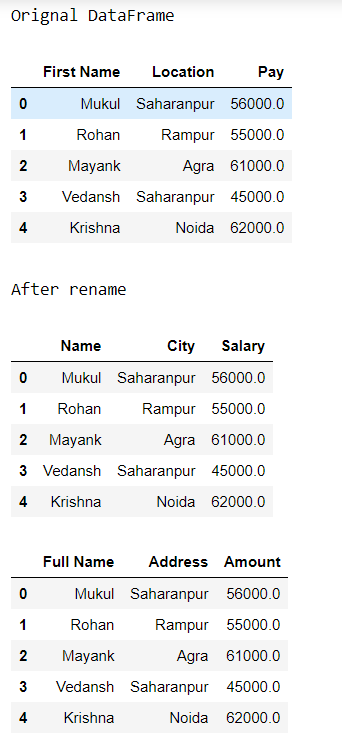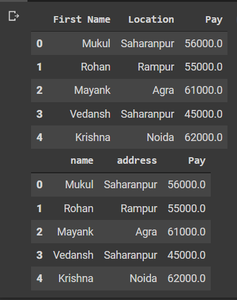# How to rename multiple column headers in a Pandas DataFrame?

Here we are going to rename multiple column headers using rename() method. The rename method is used to rename a single column as well as rename multiple columns at a time. And pass columns that contain the new values and inplace = true as an argument. We pass inplace = true because we just modify the working data frame if we pass inplace = false then it returns a new data frame.

### Way 1: Using rename() method

1. Import pandas.
2. Create a data frame with multiple columns.
3. Create a dictionary and set key = old name, value= new name of columns header.
4. Assign the dictionary in columns.
5. Call the rename method and pass columns that contain dictionary and inplace=true as an argument.

Example:

## Python

 `# import pandas` `import` `pandas as pd`   `# create data frame` `df ``=` `pd.DataFrame({``'First Name'``: [``"Mukul"``, ``"Rohan"``, ``"Mayank"``,` `                                  ``"Vedansh"``, ``"Krishna"``],` `                   `  `                   ``'Location'``: [``"Saharanpur"``, ``"Rampur"``, ``"Agra"``, ` `                                ``"Saharanpur"``, ``"Noida"``],` `                   `  `                   ``'Pay'``: [``56000.0``, ``55000.0``, ``61000.0``, ``45000.0``, ``62000.0``]})`   `# print original data frame` `display(df)`   `# create a dictionary` `# key = old name` `# value = new name` `dict` `=` `{``'First Name'``: ``'Name'``,` `        ``'Location'``: ``'City'``,` `        ``'Pay'``: ``'Salary'``}`   `# call rename () method` `df.rename(columns``=``dict``,` `          ``inplace``=``True``)`   `# print Data frame after rename columns` `display(df)`

Output:Example 2:

In this example, we will rename the multiple times using the same approach.

## Python3

 `# import pandas` `import` `pandas as pd`   `# create data frame` `df ``=` `pd.DataFrame({``'First Name'``: [``"Mukul"``, ``"Rohan"``, ``"Mayank"``,` `                                  ``"Vedansh"``, ``"Krishna"``],` `                   `  `                   ``'Location'``: [``"Saharanpur"``, ``"Rampur"``, ` `                                ``"Agra"``, ``"Saharanpur"``, ``"Noida"``],` `                   `  `                   ``'Pay'``: [``56000.0``, ``55000.0``, ``61000.0``, ``45000.0``, ``62000.0``]})`   `print``(``"Original DataFrame"``)`   `# print original data frame` `display(df)`   `# create a dictionary` `# key = old name` `# value = new name` `dict` `=` `{``'First Name'``: ``'Name'``,` `        ``'Location'``: ``'City'``,` `        ``'Pay'``: ``'Salary'``}`   `print``(``"\nAfter rename"``)` `# call rename () method` `df.rename(columns``=``dict``,` `          ``inplace``=``True``)`   `# print Data frame after rename columns` `display(df)`   `# create a dictionary` `# key = old name` `# value = new name` `dict` `=` `{``'Name'``: ``'Full Name'``,` `        ``'City'``: ``'Address'``,` `        ``'Salary'``: ``'Amount'``}`   `# call rename () method` `df.rename(columns``=``dict``,` `          ``inplace``=``True``)`   `display(df)`

Output:### Way 2: Using index

One must follow the below steps as listed in sequential order prior to landing upon implementation part as follows:

1. Import pandas.
2. Create a data frame with multiple columns.
3. The .column and .values property will return the array of columns.
4. Change the values in the array of columns using slicing.
5. Print the dataframe.

Example

## Python3

 `# import pandas` `import` `pandas as pd`   `# create data frame` `df ``=` `pd.DataFrame({``'First Name'``: [``"Mukul"``, ``"Rohan"``, ``"Mayank"``,` `                                  ``"Vedansh"``, ``"Krishna"``],` `                   `  `                   ``'Location'``: [``"Saharanpur"``, ``"Rampur"``, ``"Agra"``, ` `                                ``"Saharanpur"``, ``"Noida"``],` `                   `  `                   ``'Pay'``: [``56000.0``, ``55000.0``, ``61000.0``, ``45000.0``, ``62000.0``]})`   `# print original data frame` `display(df)`   `# renaming the column by index` `df.columns.values[``0``:``2``] ``=``[``"name"``, ``"address"` `]`   `# print Data frame after rename columns` `display(df)`

Output:Whether you're preparing for your first job interview or aiming to upskill in this ever-evolving tech landscape, GeeksforGeeks Courses are your key to success. We provide top-quality content at affordable prices, all geared towards accelerating your growth in a time-bound manner. Join the millions we've already empowered, and we're here to do the same for you. Don't miss out - check it out now!

Previous
Next